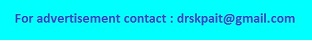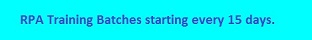# Calculate Angular and Linear Velocity

The calculator calculates the angular and linear velocity of any object moving in circular form using following formula: